Question

# 1. Show your working out for the following calculation a) TENS buffer:                              &nbsp

1. Show your working out for the following calculation

a)

TENS buffer:                                     Make from

0.1M NaOH                                        from stock 10M

0.2% SDS                                           from 10% stock

1mM EDTA                                        from 0.5M stock

10mM Tris pH 7.5                              from 1M stock

How would you make 200ml of TENS buffer from these stock solutions?

b)

5M LiCl

How would you make 5M LiCl (MW=42.40g/mol)?

c)

70% Ethanol

How would you make 1L of 70% Ethanol?

d)

HisTag Protein Purification Solutions

Charge Buffer

50mM NiSO4.6H2O

Binding Buffer

5.0mM Imidazole (MW=68.08g/mol)

0.5M NaCl (MW=58g/mol)

20mM Tris pH8

Wash Buffer

60mM Imidazole

0.5M NaCl

20mM Tris pH8

Elution Buffer

1.0M Imidazole

0.5M NaCl

20mM Tris pH8

How would you make 50ml of the Wash Buffer? (assume you have a solution of 1M Tris pH 8)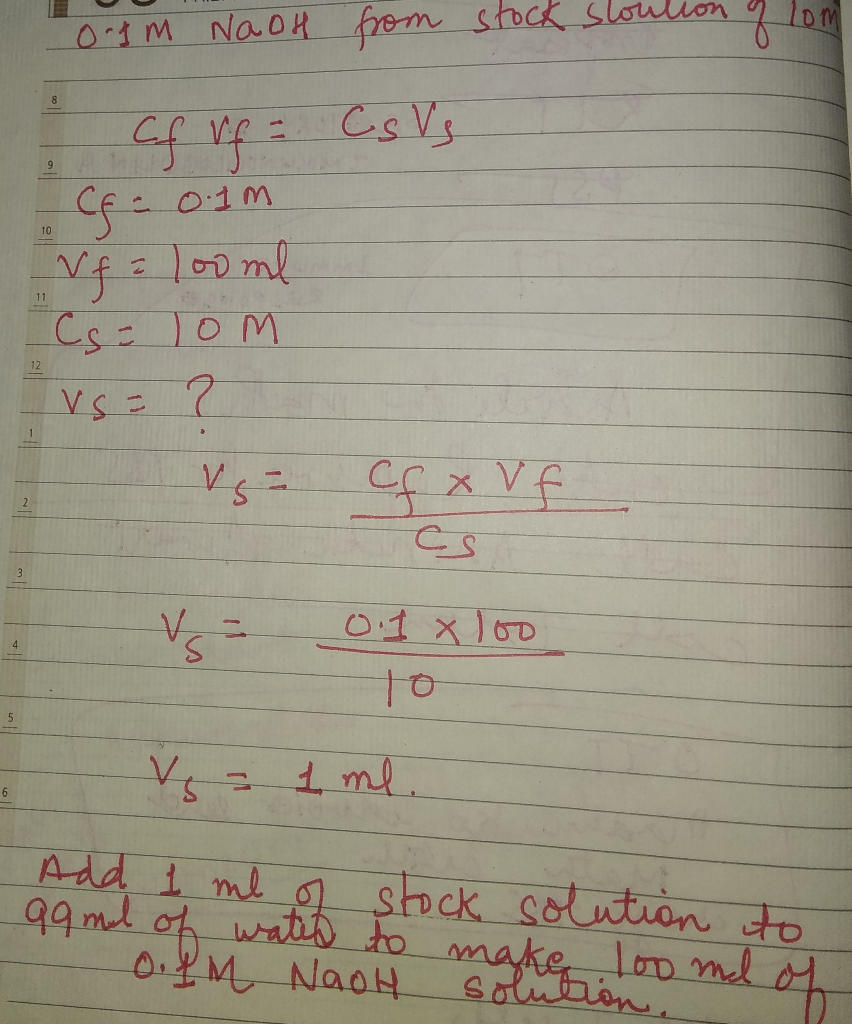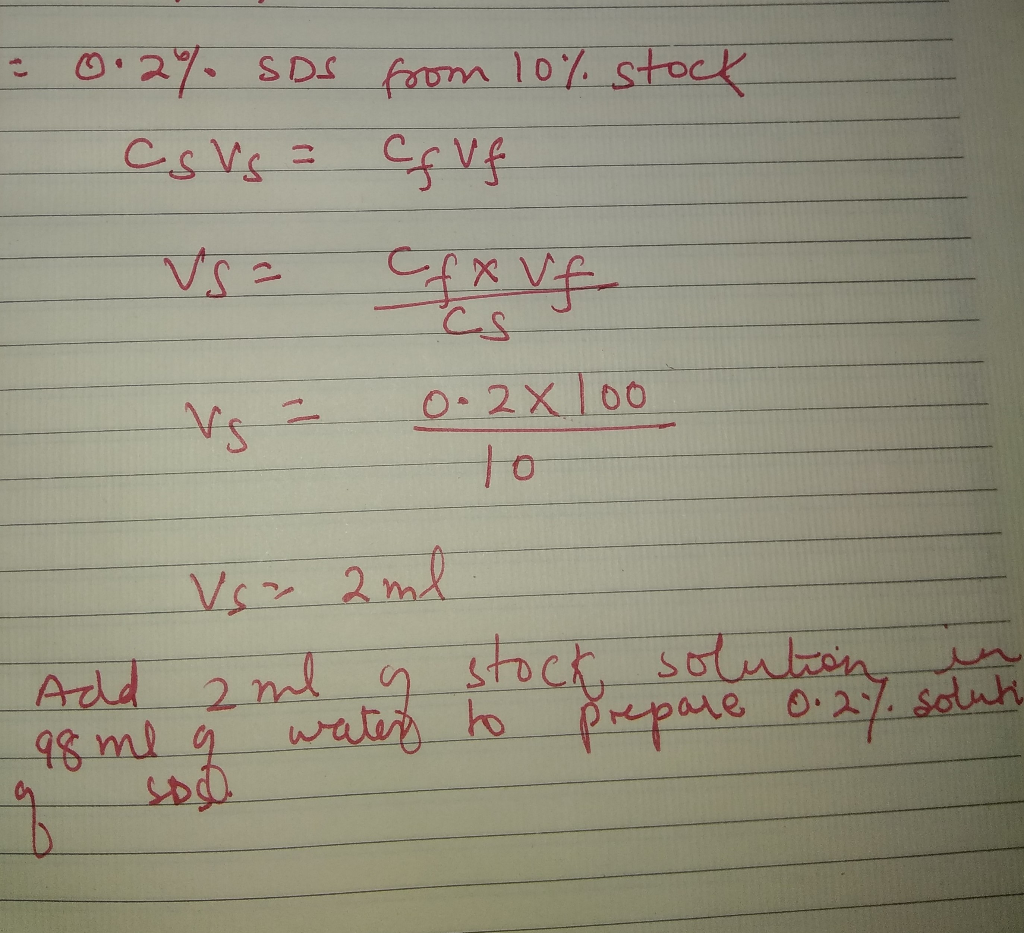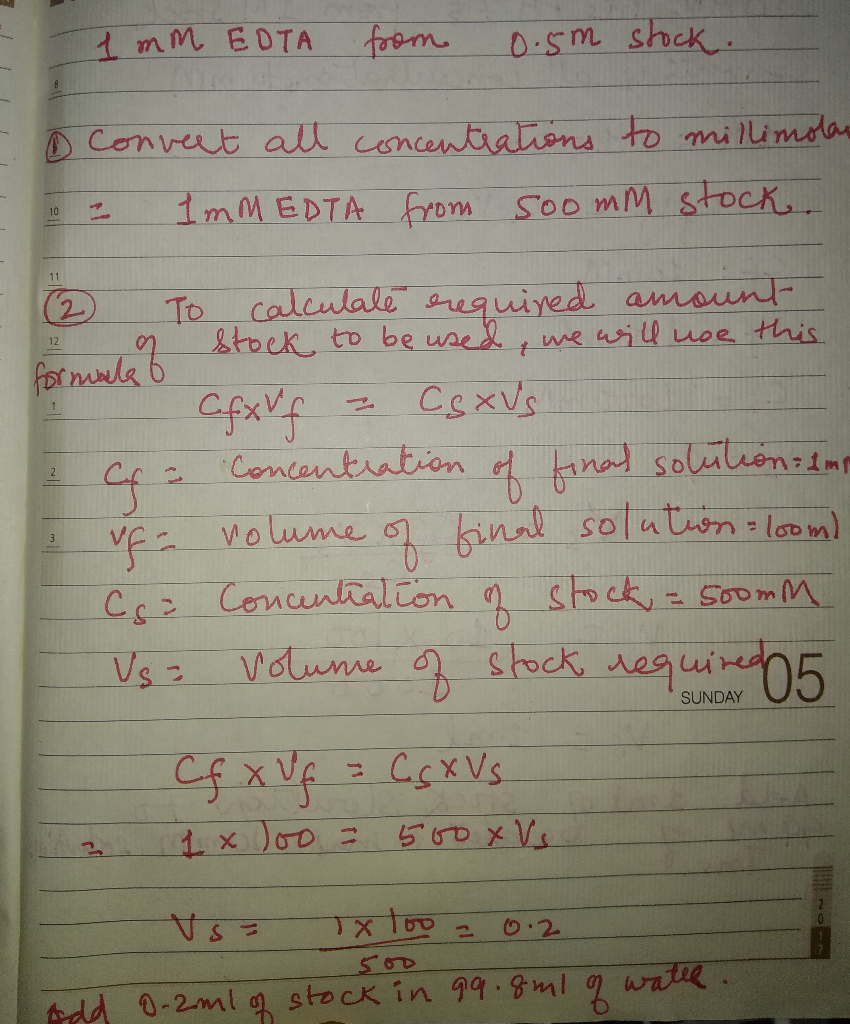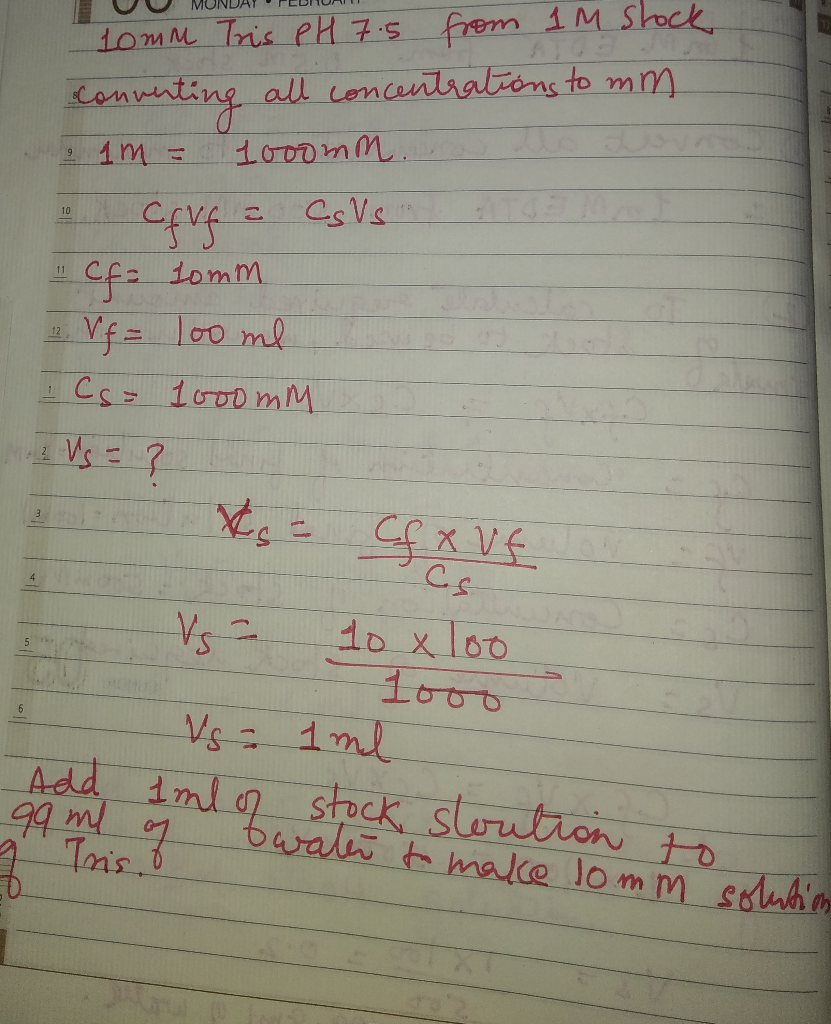TENS Buffer contains Tris, EDTA and NaOH.

1 Litre of TENS contains

10ml of 10 mM Tris-HCl

2ml of 1 mM EDTA

So for 200 ml you will require 2ml of 1M Tris-Cl pH 8 and 0.4 ml of 1 mM EDTA to be dissolved in 197.6 ml of water.

Adjust the pH of the solution using NaOH solution

#### Earn Coins

Coins can be redeemed for fabulous gifts.

Similar Homework Help Questions
• ### You must make a 1x TAE running buffer (40mM tris (pH 7.6), 20mM acetic acid and...

You must make a 1x TAE running buffer (40mM tris (pH 7.6), 20mM acetic acid and 1mM EDTA) using tris base (FW= 121.14g/mol), glacial acetic acid (17.4M) and 0.5M EDTA. show how much of each compound you would add to make 1L.

• ### Making Solutions Practice Problems: I. How would you prepare 100 ml of a 30% (w/v) solution...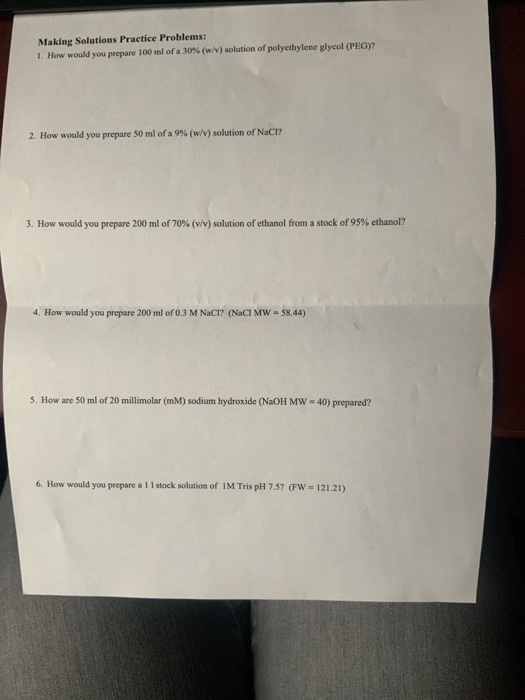Making Solutions Practice Problems: I. How would you prepare 100 ml of a 30% (w/v) solution of polyethylene glycol (PEG)? 2. How would you prepare 50 ml of a 9 % (w /v ) solution of NaCI? 3. How would you prepare 200 ml of 70 % ( v /v ) solution of ethanol from a stock of 95% ethanol? How would you prepare 200 ml of 0.3 M NaCl? (NaCl MW 58.44) 4. 5. How are 50 ml of...

• ### I need help doing problem number 5 listed below. If someone could show the work required...

I need help doing problem number 5 listed below. If someone could show the work required for this problem it would be a great help! Below is a list of solutions you will need for the mini-prep. Make 5mL of each solution as a team and store each in a 15mL Falcon tube. Store solution 1 and 70% ethanol at 4 degrees Celsius. Solution 3 and Elution Buffer can be stored at room temperature. Show your calculations in the space...

• ### completely with units and show your work for full credit. Complete on this sheet or on...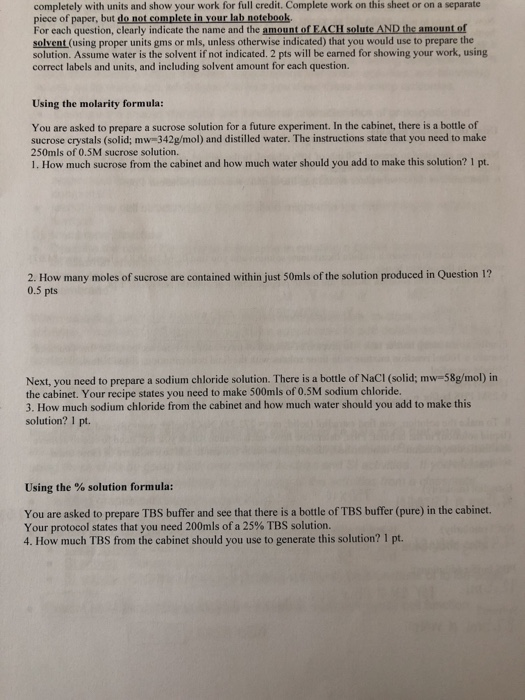completely with units and show your work for full credit. Complete on this sheet or on a separate piece of paper, but do not complete in your lab notehook For each question, clearly indicate the name and the amount of EACH solute AND the amount of solvent (using proper units gms or mls, unless otherwise indicated) that you would use to prepare the solution. Assume water is the solvent if not indicated. 2 pts will be earned for showing your...

• ### Question 7 Working with buffers You need 100 ml of 120 mM phosphate buffer and you have two stock...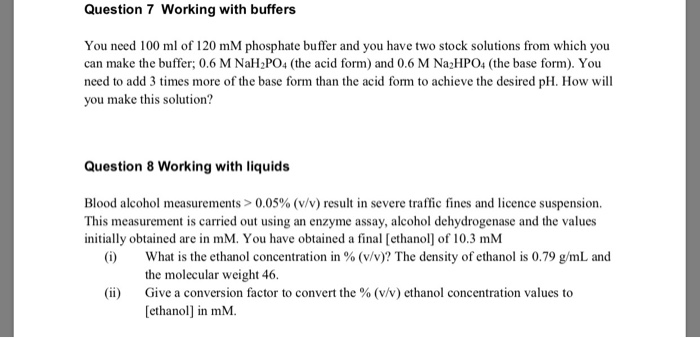please hlep me answer those three questions asap. Please ignore question 7. Question 7 Working with buffers You need 100 ml of 120 mM phosphate buffer and you have two stock solutions from which you can make the buffer; 0.6 M NaH2PO4 (the acid form) and 0.6 M Na2HP04 (the base form). You need to add 3 times more of the base form than the acid form to achieve the desired pH. How will you make this solution? Question 8...

• ### PLEASE SHOW WORK! Answers are included. What is the molarity of a 20% by weight solution...

PLEASE SHOW WORK! Answers are included. What is the molarity of a 20% by weight solution of NaOH? MW=39.99 A: 5M What percentage (%) is 1.5M NaOH? A: 6% How would you prepare 250 mL of a 0.5M NaCl solution? MW=58.44 A: 7.31g NaCl and H2O until reach 250 mL If you add 10g of NaCl to 500 mL, what is the concentration, in percent, of the solution? A: 2% NaCl solution If you add 10g of NaCl to make...

• ### Which of the following compounds would make the best buffer at pH 7.5? Acetic acid, pKa=...

Which of the following compounds would make the best buffer at pH 7.5? Acetic acid, pKa= 4.76 N-tris(hydroxymethyl) methylglycine (Tricine), pKa = 8.15 N-2-hydroxyethylpiperazine-N’-ethansulfonic acid, pKa2= 7.6 Glycine, pKa2= 9.9 Tris(hydroxymethyl)aminomethane (Tris), pKa = 8.3 I believe 3 is the correct answer and therefore I am trying to answer the below question with this information. Using the answer that you selected from question 1 above: a. Explain how you would make the buffer starting with 1M HA and 1M A-...

• ### how to solve number 2 and 3 The following chemicals have the indicated formula weights: Sucrose...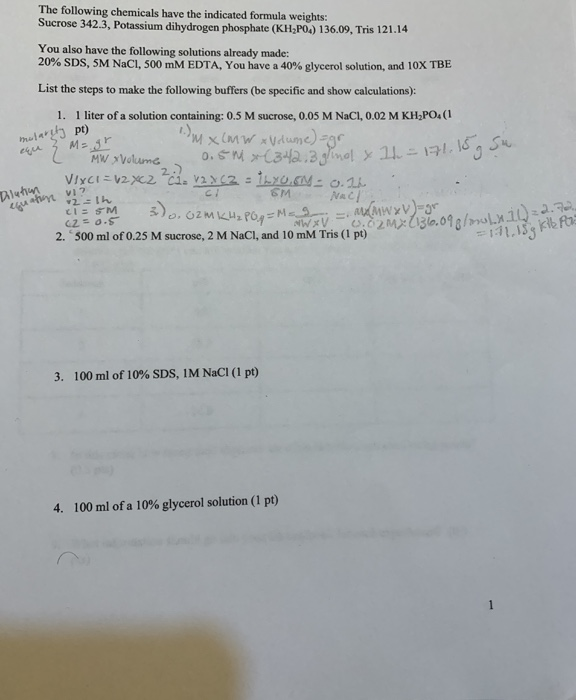how to solve number 2 and 3 The following chemicals have the indicated formula weights: Sucrose 342.3, Potassium dihydrogen phosphate (KH P04) 136.09, Tris 121.14 You also have the following solutions already made: 20% SDS, SM NaCl, 500 mM EDTA, You have a 40% glycerol solution, and 10X TBE pt) mularul Mag List the steps to make the following buffers (be specific and show calculations): 1. 1 liter of a solution containing: 0.5 M sucrose, 0.05 M NaCl, 0.02 M...

• ### You are given a stock solution of ribosylated collagen. In order to complete your experiment, you...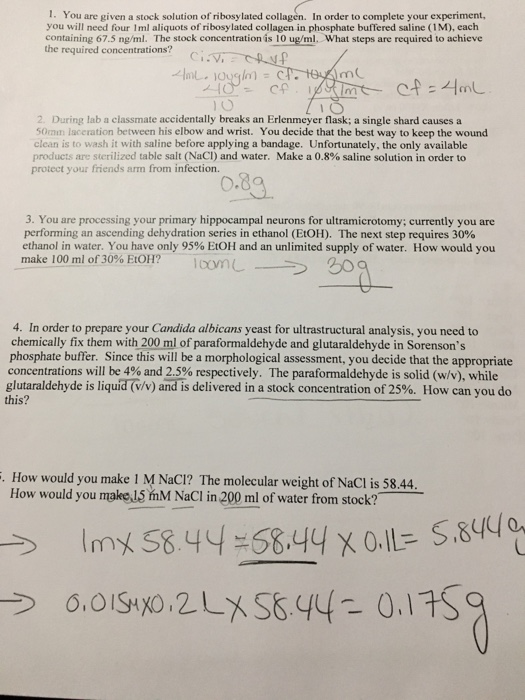You are given a stock solution of ribosylated collagen. In order to complete your experiment, you will need four 1ml aliquots of ribosylated collagen in phosphate buffered saline (1M), each containing 67.5 ng/ml. The stock concentration is 10 ug/ml, What steps are required to achieve the required concentrations? During lab a classmate accidentally breaks an Erlenmeyer flask: a single shard causes a 50mm laceration between his elbow and wrist. You decide that the best way to keep the wound clean...

• ### Solutions practice problems. calculate how much of each ingredient you would need to make the solution...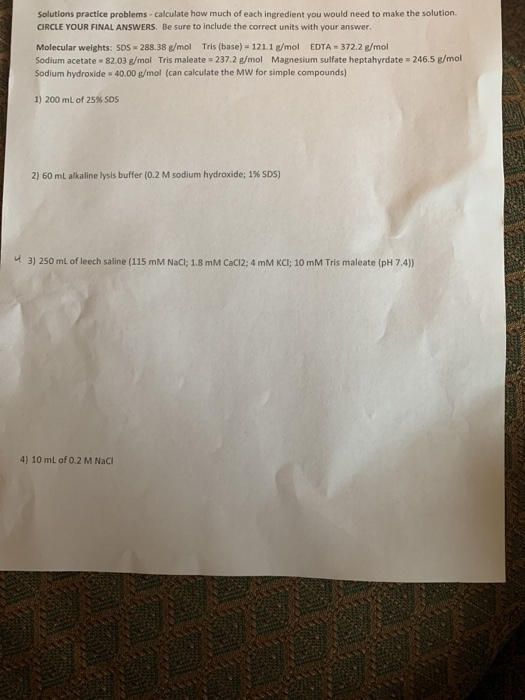Solutions practice problems. calculate how much of each ingredient you would need to make the solution CIRCLE YOUR FINAL ANSWERS. Be sure to include the correct units with your answer. Molecular weights: SDS-288.38 g/mol Tris (base) = 121.1 g/mol EDTA = 372.2 g/mol Sodium acetate 82.03 g/mol Tris maleate = 237.2 g/mol Magnesium sulfate heptahyrdate=246.5 g/mol Sodium hydroxide - 40.00 g/mol (can calculate the MW for simple compounds) 1) 200 mL of 25% SOS 2) 60 ml alkaline lysis buffer...